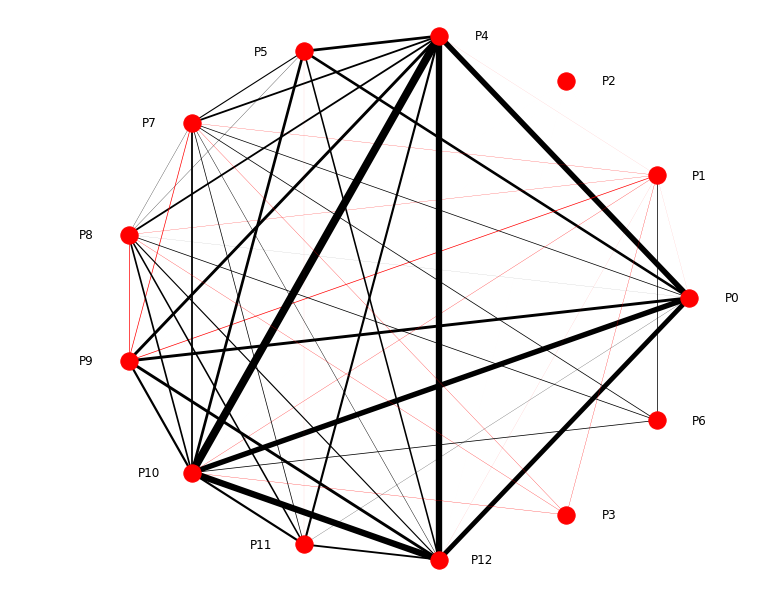# Playing around with graph renderer -- issues with edge widths and NodesAndLinkedEdges selection

I’m a relative newbie with Bokeh and I am trying to do something similar to this example – only where the widths for each edge varies with the edge weight and the colors of each edge are red if negative and black if positive. The issues I am having are:

1. The edge widths (without any interaction from clicking a node) do not all appear to line up with the actual edge weights

2. After clicking specific nodes, the width of certain edges will change, and some new edges connected to other nodes will become visible or invisible that are not supposed to (e.g., see what happens when clicking on P1, P2, P6, P12)

I’m including my code below which you may use to observe the behavior described above. I’ve also attached a pickle file which includes a dictionary which serves as input to the graph object (keys are the node pairs and values are the edge weights) and a picture of what this graph looks like when drawn using NetworkX

import pandas as pd

import numpy as np

import matplotlib.pyplot as plt

import networkx as nx

import pickle

from bokeh.io import show, output_file

from bokeh.models import Plot, Range1d, MultiLine, Circle, HoverTool, TapTool, BoxSelectTool

from bokeh.palettes import Spectral4

from bokeh.plotting import figure, reset_output

from bokeh.models import ColumnDataSource, LabelSet, StaticLayoutProvider

from bokeh.models.renderers import GraphRenderer, GlyphRenderer

with open(‘pairs.pkl’, ‘rb’) as picklefile2:

``````pairs = pickle.load(picklefile2)
``````

G = nx.Graph()

G.add_weighted_edges_from([(k, k, v) for k,v in pairs.items()])

for u,v in G.edges():

``````G[u][v]['color'] = 'red' if G[u][v]['weight'] < 0 else 'black'
``````

pos = nx.circular_layout(G)

pos_off = {}

for k, v in pos.items():

``````if v >= -.000001:

pos_off[k] = (v + 0.15, v)

else:

pos_off[k] = (v - 0.15, v)
``````

plot = figure(tools="", x_range=(-1.6, 1.6),

``````      y_range=(-1.6, 1.6), toolbar_location=None, plot_width=900, plot_height=900)
``````

node_ids = list(G.nodes())

start_ids = [a for a,b in G.edges()]

end_ids = [b for a,b in G.edges()]

weights = [.025*G[a][b][‘weight’] for a,b in G.edges()]

colors = [G[a][b][‘color’] for a,b in G.edges()]

graph_layout = pos_off

x = [v for v in graph_layout.values()]

y = [v for v in graph_layout.values()]

node_ds = ColumnDataSource(data=dict(index=node_ids,

``````                                 color=[Spectral4]*len(list(G.nodes())),

x = x,

y = y),

name="Node Renderer")
``````

edge_ds = ColumnDataSource(data=dict(start= start_ids,

``````                                  end=end_ids,

weight = weights,

color = colors),

name="Edge Renderer")
``````

graph = GraphRenderer(node_renderer=GlyphRenderer(glyph=Circle(size=15, fill_color=“color”),

``````                                              #selection_glyph=Circle(size=15, fill_color="color"),

data_source=node_ds),

edge_renderer=GlyphRenderer(glyph=MultiLine(line_alpha=0.2, line_width= 'weight', line_color = 'color'),

selection_glyph=MultiLine(line_alpha=1, line_width = 'weight', line_color = 'color'),

data_source=edge_ds),

layout_provider=StaticLayoutProvider(graph_layout=graph_layout),

``````

labels = LabelSet(x=‘x’, y=‘y’, text=‘index’, source=node_ds,

``````              background_fill_color='lightgrey')
``````

plot.xgrid.visible=False

plot.ygrid.visible=False

plot.xaxis.visible=False

plot.yaxis.visible=False

plot.renderers.append(graph)

plot.renderers.append(labels)

show(plot)

``

Let me know if any additional information is needed and if the code above is particularly unclear. Thanks for any help/insight in advance!

pairs.pkl (1.69 KB)